RAGGIO'S ELECTROMAGNET DESIGN COOKBOOK

#### This is a simple approach to practical electromagnet designs, I avoid all unnecessary theory, assuming that the reader have some basics concepts in electrical and magnetic circuit theory. I have no considerations for the dispersed flux in the iron near the air gap.

The use of ( ), [ ], *, simbols are for help in clarity of equations.

```                           INDEX

1-Some theoretical considerations.
2-Practical electromagnet for practical example.
3-General considerations.
4-Practical design by the BRUTE-FORCE method.
5-Practical circuit by BRUTE-FORCE method.
6-Practical design by a REFINED method.
7-practical circuit by REFINED method.

----------------------------------------------------------------------------

1- SOME TEORETICAL CONSIDERATIONS

air-gap
|
-------------------    -------------------
| iron core       |    |     li          |
|   ............. | la |....<..>......   |
|   .             |    |             .   |
|   .   -----------    -----------   .   | la=longitude of air gap
|   .   |                        |   .   | li=mean longitude of iron
|   .   |                        |   .   |
|   .   |                        |   .   |
|   .   |                        |   .   |
|   .   |                        |   .   |
|   .   |                        |   .   |
|   .   |                        |   .   |
|   .   ------/\ -------/\-------    .   |
|   .        /  \      /  \          .   |
|   ......../....\.. ./....\..........   |
|          /      \  /      \            |
----------/--------\/--------\------------
|                  |
|                  |
|                  |
Coil of N turns, circulating I current

FIG 1. Typical magnetic circuit with iron core and air-gap

1.1-MAGNETOMOTIVE FORCE
-----------------------
In Fig 1 the magnetic flux is caused by N turns of wire carrying a
current of I amperes and producing a magnetomotive force given by:

F= (N * I) / 0.796  (1)             (* denotes multiplication sign)

were:

F: magnetomotive force in ampere.turn
N: number of turns
I: current in amperes

1.2 MAGNETIZING FORCE
--------------------
The magnetizing force is defined as the magnetomotive force per
unit length of path:

H=F/l    (2)

were:

H:magnetizing force in LENZ
F:magnetomotive force in amper.turn
l:length of path in cm.

1.3 PERMEABILITY
----------------
The permeability (Mu) is defined by the relationship:

Mu=B/H    (3)

were:

Mu:Permeability
B:Flux density, in GAUSS
H:Magnetizing force in  Lenz
In the air, H is numerically equal to the flux density B

Permeability is the equivalent of conductivity in electrical circuits.
Permeability in iron cores is not constant, but varies when the flux
is varied. The relationship between B, H and Mu is shown by the
"BH caracteristic curve" of the iron, as shown in FIG 2. and  photos 2 and 3.
The value of Mu at any point is the value  of B divided by the value of H at
that point.
Generally the iron materials have hight Mu values, this implicates
that the iron have hight conductivity, or low resistance to the
magnetic flux; iron is a good "magnetic conductor".
In the opposite side, the air  have low conductivity, and hence hight
resistance; air is a bad "magnetic conductor".

B
|
|             * *  *
|          *
|        *
|       *
|      *
|     *
|    *
|   *
|*____________________
H
FIG 2.  BH caracteristic plot of iron.

```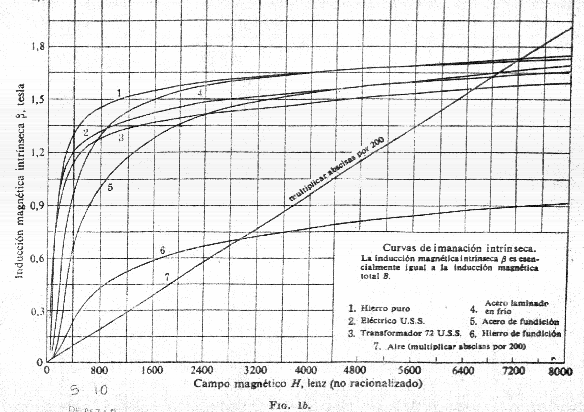Photo 2.- B-H iron curves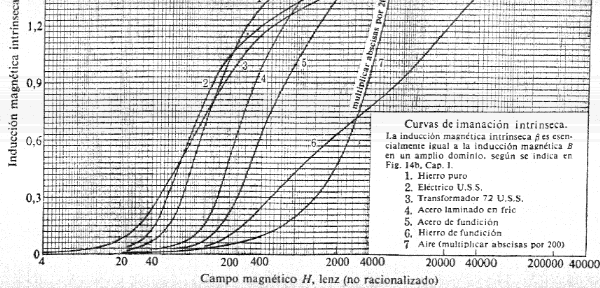Photo 3.- B-H iron curves, detailed for low H
```2- PRACTICAL ELECTROMAGNET FOR EXAMPLES

Electromagnet  to generate a field strength of 1174 gauss in a gap
of 2 cm.
The square  section of the iron is 5 x 5 cm.
The coil of the magnet have 720 turns of 1.5 mm wire. The coil length
is 12 cm.

/--------------- 22.5 cm ----------------/

/-----------------/    /--------------- -/|     ---
/                 /    /                 /  |      |
-------------------    -------------------    |      |
|                 |    |     li          |    |      |
|   ..............| la |............ .   |    |      |
|   .             |    |             .   |    |      |
|   .   -----------    -----------   .   |    |      |
|   .   |                        |   .   |    |      |
|   .   |                        |   .   |    |     15 cm
|   .   |                        |   .   |    |      |
|   .   |                        |   .   |    |      |
|   .   |  ********************  |   .   |    |      |
|   .   |  ******* COIL *******  |   .   |    |      |
|   .   |  ********************  |   .   |    |      |
|   .   --------------------------   .   |    |      |
|   .                                .   |    |      |
|   ..................................   |   /      ---
|                                        | /
------------------------------------------
********************
******* COIL *******
********************
FIG 3. Phisical dimensions of a practical electromagnet used for
the examples. this was my first electomagnet I buid.

```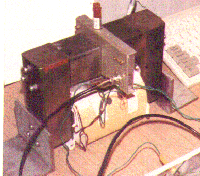Photo 1.- First electromagnet for NMR experiments, show with probehead in the air-gap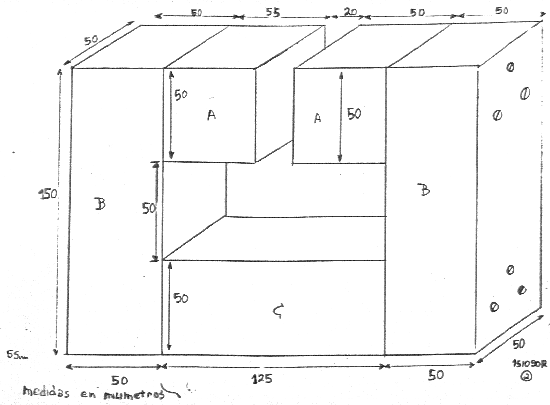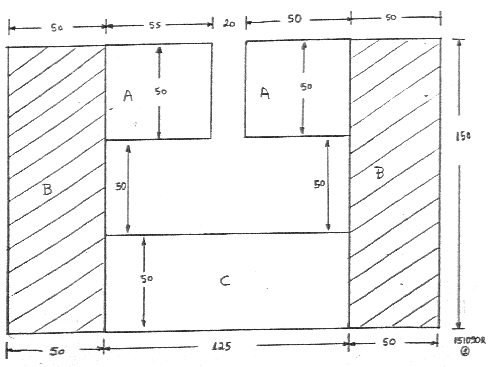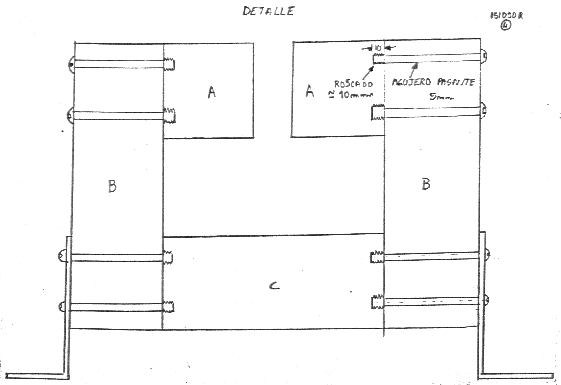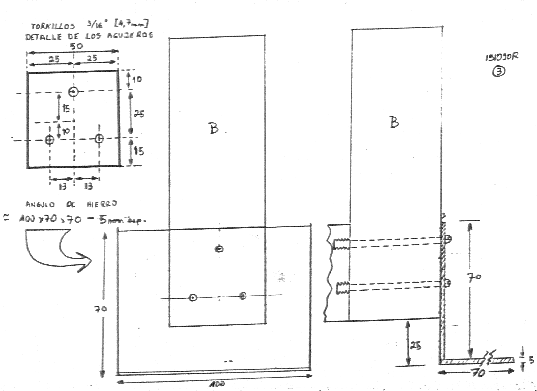Scaned schematics of the electromagnet showing construction details

```3-GENERAL CONSIDERATIONS

The following assumptions are generally made for simple theoretical
treatment of circuit of FIG 1:

3.1 In the magnetic circuit, we assume that all necessary
magnetomotive force is generated  to overcome the air-gap
"resistance".

3.2 The flux is confined itself entirely to the iron over the whole
length of the iron path.

3.3 That the flux is uniformly distributed over the cross-sectional
area of the iron.

3.4  That the iron area is the same along the magnetic circuit.

3.5 That the air gap length is small compared to the poles area.

3.6 That the gap area is the same as the iron area.

4- PRACTICAL DESIGN by BRUTE-FORCE method

Target:
Find the NI (amper.turn) necessary to generate a desired flux
density (B) in a  determined air gap (la).

Solution:
In the magnetic circuit of FIG 1 we can assume that in the gap region,
the magnetic induction is uniform, then:

H=B     (4)

H expresed in ampere.turn/cm
B expresed in gauss

Then we can assume that all magnetomotive force is necessary to overcome
the gap resistance:

of eq. (2):       H=F/la

of eq. (4):       H=B

Then:             B=F/la

Finally:          F=B * la      (5)

Remember:         F= (N * I) / 0.796   from eq. (1)

Then  (5)=(1)

B * la= (N * I) / 0.796

Finally           N * I= 0.796 * B * la         (6)

were:
N * I: is in ampere.turn
B:     is in gauss
la:    is in centimeters

NOTE: This BRUTE-FORCE method do not involve the dimensions of the
magnetic circuit, except the AIR-GAP length.
However, as a first aproximation have good results between +/- 10 %.

5- PRACTICAL CIRCUIT using the BRUTE-FORCE method.

Example:
For the NMR experiments, is needed a B of 1174 gauss in an air gap
of 2 cm.
Then, the NI necessary are:

from eq. (6):

N * I = 0.796 * B * la

replacing values:

N * I = 0.796 * 1174 * 2 = 1869

-----------------
N * I = 1869
-----------------

Then, if we have a coil with 1869 turns, there  must circulate one ampere of
current to generate the necessary field in the gap.
The design of the coil is out of this paper.
Having built the electromagnet, from the practical circuit, we have
the following values measured:

magnetic field of 1174 gauss   (measured with NMR signal)
coil of 720 turns
current of 2.76 amperes   (measured with digital ammeter 3-1/2 digits)

then N * I= 720 * 2.78 = 2001.6

This experimental value is between +7% of the theorical value.
Photo 1 shown the electromagnet.

6- PRACTICAL DESIGN by A REFINED METHOD

In this method, we use  the dimensions of the iron circuit, and the
B-H curves of the iron (photos 2 and 3).

Target:
Find the N*I necessary to generate a desired B in a determined
air-gap.

Solution:
In the magnetic circuit of FIG. 1  we can assume that in the gap region
the magnetic induction is uniform, then:

from eq. (4)               H=B

Then we can calculate the total magnetomotive force, including
now the iron length:

F = Ha * la + Hi * li       (7)

from eq. (3)

H= B/Mu

Then replacing H:

F= (Ba/Mua) * la + (Bi / Mui) * li

The Mu of air, Mua=1, then:

F = Ba * la + (Bi / Mui) * li

assuming Ba=Bi :

F= B * [ la + (li / Mui)]       (8)

from eq. (1)     F= (N * I) / 0.796

Then (1)=(8)

(N * I) / 0.796 = B * [la + (li / Mui) ]

Finally:

---------------------------------------
N * I  = B * 0.796 * [la + (li / Mui) ]
---------------------------------------

The value of Mui must be obtained from the B-H curves of the iron, Photo 2 and 3.

7- PRACTICAL CIRCUIT by the REFINED method.

Verification of the practical circuit:
From FIG 3:

B=1174 gauss
la= 2cm
li= 54 cm
Mui= 2730 from the B-H curves, see photo  3.

For this example, for B=1174 correspond a H of 35 Lenz, which correspond
to 0.43 Oersted, curve 2 of photo 3. (See at end the  conversion  tables
between units).
These values obtained graphically, are approximated.
Then Mui=1174/0.43=2730

replacing values:

N * I = 1174 * 0.796 * [( 2 + (54 / 2730) ]

N * I = 934.50 * [ 2 + 0.01978 ]

---------------
N * I = 1887.4
---------------

Then  the refined method results: N * I = 1887
remember the brute force results: N * I = 1869
and the practical measurement:    N * I = 2001

This results (refined) is near the -7 % of the practical measurement.

As you can see, the "resistance" of the iron path is very low, as
compared to the "resistance" of the air-gap. Then, all the necessary N * I
generate is employed  to  overcome the air-gap "resistance".

Then this results arises that since refined method is only a little more
exact and  the BRUTE-FORCE method is preferible, for most practical designs.
Both methods give results below the practical measurements between 7-8%.

-------------------------------------------------------------------------------------------------------

Conversion between electromagnetics units

Multiply                   BY                     To get

F  in uem                     10               F  in ampere.turn
F  in Gilbert                0.7958            F  in ampere.turn
F  in ampere.turn            1.257             F  in Gilbert

H  in Oersted               79.58              H  in Lenz
H  in Lenz                   0.01257           H  in Oersted
H  in Oersted                2.02              H  in ampere.turn/inch
H  in ampere.turn/inch       0.495             H  in Oersted

B  in Gauss                 0.0001             B  in Tesla
B  in Tesla                 10000              B  in Gauss
B  in Gauss                  1                 B  in  Maxwells/sq.cm
B  in Gauss                  6.45              B  in Maxwells/sq.inch
B  in Tesla                  1                 B  in Weber/sq.metre

------------------------------------------------------------------------------------------------------------

Bibliograpy:

RCA-Radiotron Designer's Handbook, 4 th. edition, 1953

Circuitos Magneticos y Transformadores- EE Staff del M.I.T. , 1965
Editorial Reverte, Spain.

Editorial Arbo, Argentina.

-----------------------------------------------------------------------------------------------------------

FINAL NOTE;

This design of electromagnets, was totally secondary to my main work in NMR,
and was made only because I do not had any electromagnet  at the time I begin
to experiment in low resolution NMR techniques.
Probably, someone with good background in physics or in electromagnet
design can made some observation in this paper, it will be welcome.

------------------------------------------------------------------------------------------------------------

Norberto Raggio's Electromagnet Design Cookbook
Writed by Norberto Raggio,
Buenos Aires,
Argentina.

e-mail:  raggio@technologist.com
http://www.geocities.com/CapeCanaveral/2404/97&keywords=KEY=CapeCanaveral", "w3adJIQJAAII", "width=515,height=125"); //-->